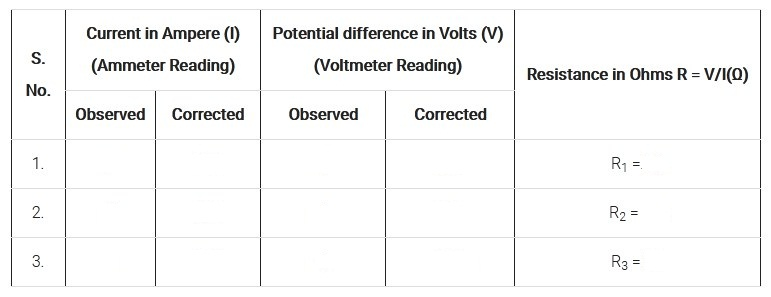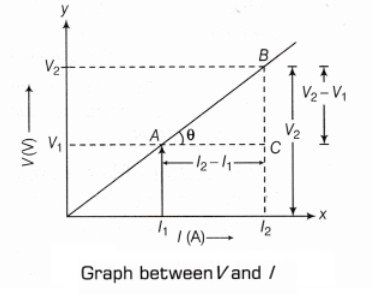# To Verify the Ohms Law & Plot V-I Characteristics

## Aim of the Experiment

To study the dependence of potential difference (V) across a resistor on the current (I) passing through it and determine its resistance (R). Also plot a graph between V and I

##### Apparatus Required

1. Voltmeter of suitable range
2. Ammeter of suitable range
3. Resistance wire or Resistor
4. Connecting wires
5. A Key
6. A Dry cell (or battery eliminator)
7. Rheostat
8. A piece of sand paper

### Theory / Principle

The dependence of current on potential difference can be studied by Ohm?s law. According to Ohm?s law, the potential difference (V) across the ends of a resistor is directly proportional to the current (I) through it provided its temperature remains the same.
i.e. V ∝ I
or; V / I = Constant = R
or; V = RI

Here, R is a constant for the given resistor at a given temperature and is called its resistance. The SI unit of resistance is ohm (Ω).
The graph between the potential difference across the two ends of a resistor and the current passing through it is a straight line passing through the origin. The slope of this straight line graph gives the resistance R of the resistor

### Procedure

1. Before starting the experiment, note down the range, least count and zero error (if any) of the ammeter and voltmeter.

2. Wipe the ends of the connecting wires with sand paper to remove any insulating layer.

3. Make a set up of the circuit by connecting different components with the help of connecting wires as shown in the circuit diagram below:4. Connect the key, the rheostat, the ammeter and the resistor in series with the connecting wires to the dry cell, as shown in the circuit diagram, Ensure that positive (+) terminal of ammeter is joined to the positive (+) terminal of the cell. Make neat and tight connections.

5. Connect the voltmeter in parallel to the resistor, as shown in circuit diagram. Ensure that the positive (+) terminal of the voltmeter is joined to the positive (+) terminal of the cell, and the negative (-) terminal of the voltmeter is joined to the negative (-) terminal of the cell.

6. Insert the key in the plug to let the current establish in the circuit.

7. Adjust the rheostat, so that a small current passes through the resistor, say 0.1 A, so the reading of ammeter will be 0.1 A.

8. Read the corresponding value of potential difference from voltmeter.

9. Repeat the experiment by adjusting the slider, for the values of current 0.3A, 0.4A, 0.5A etc. and record the corresponding values of potential difference in voltmeter.

10. Lastly, plot a graph between V and I taking V along y-axis and I along x-axis.

### Observation

Range of ammeter = ......... A
Least count of ammeter = ........... A
Zero error of ammeter = ........... A
Range of voltmeter = ........... V
Least count of voltmeter = ......... V
Zero error of voltmeter = ............ V### Calculation

Mean value of resistance = (R1 + R2 + R3 + R4)/4 = ............ Ω

Now, by plotting the graph between V and I taking V along y-axis and I along x-axis. We get a straight line and the slope of V versus I graph gives the resistance of the resistor.

i.e. tanθ = V/I = R = Resistance

Therefore; Slope = BC/AC = (V2 ? V1)/(I2 ? I1) = R### Result

1. From the above calculation and observation, we have concluded that for a resistor, if temperature remains constant then current through the resistor is directly proportional to the potential difference applied across it.

2. The value of resistance R of resistor remains the same for all values of current through it. The graph between V and I is a straight line and passes through the origin. This verifies Ohm?s law.

### Precautions

1. Wires should be thick and their insulation of ends should be removed properly.
2. All the connections should be tight otherwise some external resistance may introduce in the circuit.
3. Ammeter must be connected in series with the circuit.
4. Voltmeter must be connected in parallel with the circuit.
5. Zero mark of ammeter and voltmeter should be checked properly.
6. There should be tight connections of connecting wires.
7. Put the plug in key, only when reading is to be recorded. Take off plug immediately after it, to avoid unnecessary heating of wires.
8. Positive terminals of voltmeter and ammeter should be connected to positive terminal of the cell and vice-versa.

### Sources of errors

• There may be a reading error of ammeter and voltmeter.
• Area of cross-section of wire may not be uniform.
• It may be possible that current has flown for longer period of time through the circuit.
• There may be a loose connection in electrical circuit.

### Viva Questions and Answers for Ohms law Experiment

Question.1: In this experiment, it is advised to take out the key from the plug when the observations are not being taken. Why?
Answer: It is to avoid the unnecessary heating of wires. Heating may change the resistance of resistors.

Question.2: Suppose the ammeter (or voltmeter) you are using in this experiment do not have positive (+) and negative (-) terminal markings. How will you use such ammeter (or voltmeter) in the circuit?
Answer: First of all we connect ammeter or voltmeter arbitrary in the circuit, if deflection occurs in opposite direction. It means the connection is not proper. Now by interchanging the terminals, we can use it in the circuit.

Question.3: Why is it advised to clean the ends of connecting wires before connecting them?
Answer: Ends of wire should be cleaned to remove the insulation (or insulating layer) of dust particles since, presence of insulation (which is a non- conducting material) wilt not allow current to flow in the circuit.

Question.4: What do you mean by a battery eliminator?
Answer: A device which is used in place of battery or cells, is known as a battery eliminator.

Question.5: What are the necessary conditions for current flow through the conductor?
Answer: There are two such conditions: there must be a closed circuit and there must be a source of electrical energy.

Question 6: Can you explain why there is no current in the circuit, if plug is removed?
Answer: As we know air gap gives infinite resistance to the circuit. So, if the plug is removed, there will be no current in the circuit.

Question 7: What do you understand by the term resistance of a conductor?
Answer: The resistance of a conductor may simply be explained as being the obstruction offered to the passage of electric current by the conductor in the circuit.

Question 8: What do you understand by the term emf of a cell?
Answer: The maximum potential difference which exists across the terminals of a cell when no current is drawn out of the cell is called emf (electromotive force) of a cell.

Question 9: What do you understand by the term terminal potential difference of a cell?
Answer: The potential difference which exists across the terminals of a cell when current is being drawn from the cell, is known as terminal potential difference of a cell.

Question 10: What is the composition of nichrome alloy?
Answer: The composition of nichrome alloy are as follows: Ni = 68%, Cr = 15%, Fe = 15.5%, Mn = 1.5%

## Related Categories### Spreading Knowledge Across the World

USA - United States of America  Canada  United Kingdom  Australia  New Zealand  South America  Brazil  Portugal  Netherland  South Africa  Ethiopia  Zambia  Singapore  Malaysia  India  China  UAE - Saudi Arabia  Qatar  Oman  Kuwait  Bahrain  Dubai  Israil  England  Scotland  Norway  Ireland  Denmark  France  Spain  Poland  and  many more....